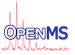OpenMS

#include <OpenMS/CHEMISTRY/AdductInfo.h>

[legend]

## Public Member Functions

double getNeutralMass (double observed_mz) const
returns the neutral mass of the small molecule without adduct (creates monomer from nmer, decharges and removes the adduct (given m/z of [nM+Adduct]/|charge| returns mass of [M]) More...

double getMZ (double neutral_mass) const
returns the m/z of the small molecule with neutral mass neutral_mass if the adduct is added (given mass of [M] returns m/z of [nM+Adduct]/|charge|) More...

double getMassShift (bool use_avg_mass=false) const
returns the mass shift caused by this adduct if charges are compensated with protons More...

bool isCompatible (const EmpiricalFormula &db_entry) const

int getCharge () const

const StringgetName () const
original string used for parsing More...

const EmpiricalFormulagetEmpiricalFormula () const
sum formula of adduct itself. Useful for comparison with feature adduct annotation More...

UInt getMolMultiplier () const
get molecular multiplier More...

bool operator== (const AdductInfo &other) const
equality operator More...

## Private Attributes

String name_
members More...

EmpiricalFormula ef_
Sum formula for the actual adduct e.g. 'H' in 2M+H;+1. More...

double mass_
computed from ef_.getMonoWeight(), but stored explicitly for efficiency More...

int charge_
negative or positive charge; must not be 0 More...

UInt mol_multiplier_
Mol multiplier, e.g. 2 in 2M+H;+1. More...

## Constructor & Destructor Documentation

 AdductInfo ( const String & name, const EmpiricalFormula & adduct, int charge, UInt mol_multiplier = 1 )

C'tor, to build a representation of an adduct.

Parameters
 name Identifier as given in the Positive/Negative-Adducts file, e.g. 'M+2K-H;1+' adduct Formula of the adduct, e.g. '2K-H' charge The charge (must not be 0; can be negative), e.g. 1 mol_multiplier Molecular multiplier, e.g. for charged dimers '2M+H;+1'

## ◆ getCharge()

 int getCharge ( ) const

## ◆ getEmpiricalFormula()

 const EmpiricalFormula& getEmpiricalFormula ( ) const

sum formula of adduct itself. Useful for comparison with feature adduct annotation

## ◆ getMassShift()

 double getMassShift ( bool use_avg_mass = false ) const

returns the mass shift caused by this adduct if charges are compensated with protons

Referenced by NucleicAcidSearchEngine::main_().

## ◆ getMolMultiplier()

 UInt getMolMultiplier ( ) const

get molecular multiplier

## ◆ getMZ()

 double getMZ ( double neutral_mass ) const

returns the m/z of the small molecule with neutral mass neutral_mass if the adduct is added (given mass of [M] returns m/z of [nM+Adduct]/|charge|)

## ◆ getName()

 const String& getName ( ) const

original string used for parsing

## ◆ getNeutralMass()

 double getNeutralMass ( double observed_mz ) const

returns the neutral mass of the small molecule without adduct (creates monomer from nmer, decharges and removes the adduct (given m/z of [nM+Adduct]/|charge| returns mass of [M])

## ◆ isCompatible()

 bool isCompatible ( const EmpiricalFormula & db_entry ) const

checks if an adduct (e.g.a 'M+2K-H;1+') is valid, i.e. if the losses (==negative amounts) can actually be lost by the compound given in db_entry. If the negative parts are present in db_entry, true is returned.

## ◆ operator==()

 bool operator== ( const AdductInfo & other ) const

equality operator

static

parse an adduct string containing a formula (must contain 'M') and charge, separated by ';'. e.g. M+H;1+ 'M' can have multipliers, e.g. '2M + H;1+' (for a singly charged dimer)

## ◆ charge_

 int charge_
private

negative or positive charge; must not be 0

## ◆ ef_

 EmpiricalFormula ef_
private

Sum formula for the actual adduct e.g. 'H' in 2M+H;+1.

## ◆ mass_

 double mass_
private

computed from ef_.getMonoWeight(), but stored explicitly for efficiency

## ◆ mol_multiplier_

 UInt mol_multiplier_
private

Mol multiplier, e.g. 2 in 2M+H;+1.

## ◆ name_

 String name_
private

members

arbitrary name, only used for error reporting